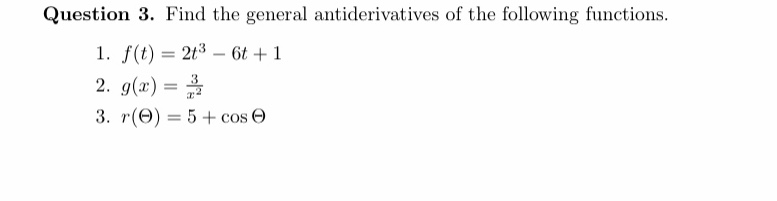Question 3. Find the general antiderivatives of the following functions.1. f(t) 23 6 12. g)3. r(e)-5+cos

Question

How do you solve #1help_outlineImage TranscriptioncloseQuestion 3. Find the general antiderivatives of the following functions. 1. f(t) 23 6 1 2. g) 3. r(e)-5+cos fullscreen
Step 1

We have to find general antiderivative of f(t) where f(t) is given by

Step 2

General antiderivative is represented by I.

we will use following formula to find antiderivative.

Step 3

With the help of above power rule we will fin...

Want to see the full answer?

See Solution

Want to see this answer and more?

Our solutions are written by experts, many with advanced degrees, and available 24/7

See Solution
Tagged in

Integration